# Veggie maths Mash: Two-Digit Subtraction

Make maths palatable with these food-themed puzzles. With all of those veggies featured you'll be giving your kid some essential maths practise and encouraging healthy eating habits at the same time.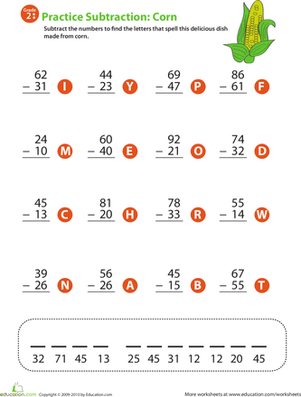### 2-Digit Subtraction

Give your child's maths skills a boost with this year 3 subtraction worksheet that includes two-digit problems and a word puzzle at the end!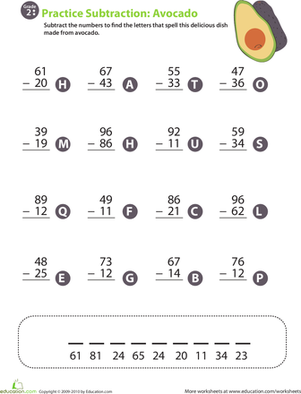### Veggie maths Mash: Two-Digit Subtraction 2

Give your child valuable at-home maths practise with this year 3 worksheet that includes two-digit subtraction problems and a word puzzle at the end.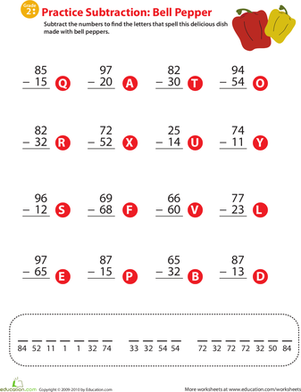### Veggie maths Mash: Two-Digit Subtraction 3

Satisfy your child's craving for maths with this veggie-themed year 3 worksheet that offers two-digit subtraction problems and a word puzzle at the end.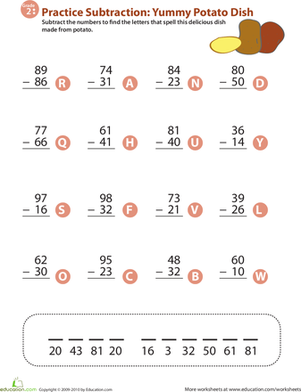### Veggie maths Mash: Two-Digit Subtraction 4

Offering 16 two-digit subtraction problems and a word puzzle at the end, this year 3 maths worksheet provides a healthy dose of mental nutrition.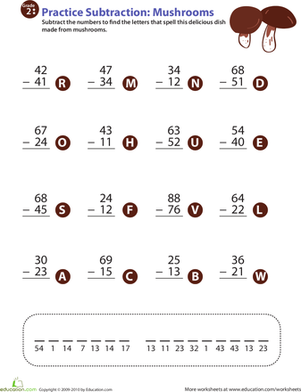### Veggie maths Mash: Two-Digit Subtraction 5

Improve your second grader's subtraction facts fluency with this mushroom-themed maths worksheet that ends with a surprise: a word puzzle!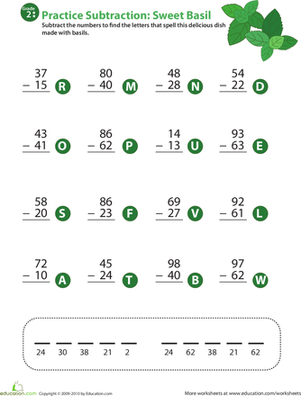### Veggie maths Mash: Two-Digit Subtraction 6

Help your second grader learn two-digit subtraction with this maths worksheet that offers 16 subtraction problems and a fun word puzzle at the end.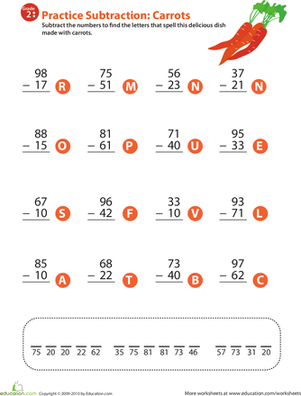### Veggie maths Mash: Two-Digit Subtraction 7

Carrots add fun and interest to this year 3 maths worksheet that helps your child learn his subtraction facts and hone his mental maths ability.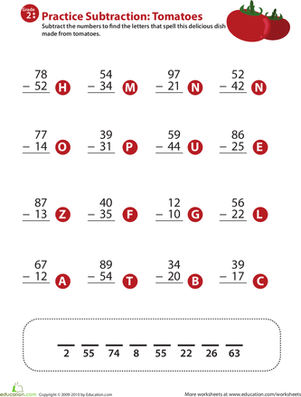### Veggie maths Mash: Two-Digit Subtraction 8

To help improve your child's subtraction facts fluency, download this cheery year 3 worksheet that offers 16 subtraction problems and a fun tomato theme.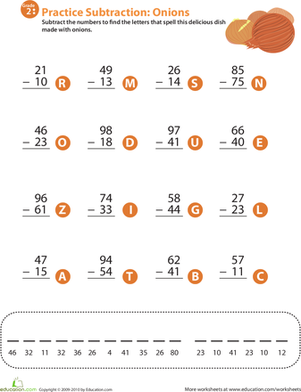### Veggie maths Mash: Two-Digit Subtraction 9

This year 3 maths worksheet makes subtraction fun. Children complete the two-digit problems, then use their answers to solve a word puzzle.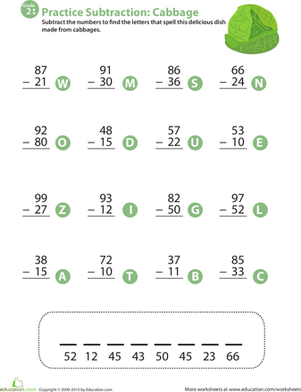### Veggie maths Mash: Two-Digit Subtraction 10

Explore the mathematical side of cabbage in this year 3 worksheet that combines two-digit subtraction practise with a cabbage-themed word puzzle.

Create new collection

0

### New Collection>

0Items

What could we do to improve Education.com?